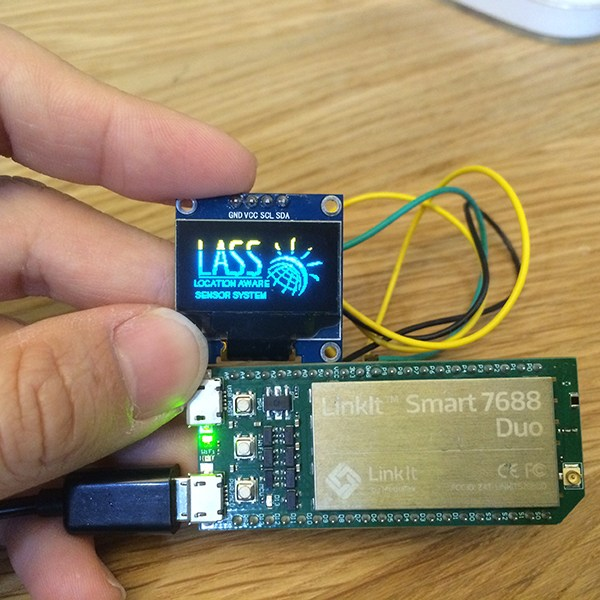# 用7688 Duo遠端控制PM2.5偵測裝置

Home All用7688 Duo遠端控制PM2.5偵測裝置## 用7688 Duo遠端控制PM2.5偵測裝置

### 必備物品

• Mediatek LinkItSmart 7688 Duo x1
• SSD1306相容的128 x 64 I2C OLED模組 x1

### 選配物品(控制空氣清淨機)

1. 5V Relay 模組  x1
2. 電力配線用一連明盒附插座  x1
3. 有插頭的多芯電線  x1

### 設計步驟二：取得，並測試線上正在運作的各種資料

LASS的MQTT伺服器位址是 「gpssensor.ddns.net」，服務Port一樣是 「1883」，填寫完後點擊右下角的「Create Connection」。

``|ver_format=3|fmt_opt=0|app=PM25|ver_app=0.7.13|device_id=FT1_004|tick=260989326|date=2016-01-21|time=06:27:23|device=LinkItONE|s_0=67852.00|s_1=100.00|s_2=1.00|s_3=0.00|s_4=662.00|s_d0=66.00|s_t0=21.40|s_h0=72.50|s_d1=81.00|gps_lat=23.284105|gps_lon=120.275816|gps_fix=1|gps_num=16|gps_alt=5``

### 設計步驟四：於Linux端取得MQTT資料分封傳送

LASS的Github上，已經有陳伶志博士提供：MQTT資料轉送到Thingspeak的程式，只要將它進行稍微的修改就可以達到本次需要的目標了，開源專案的最大好處就是如此，站在巨人的肩膀上，輕輕一跳大家就可以到更高的地方！

``pip install pyserial paho-mqtt``

``scp lass-pm25-friend.py root@mylinkit.local:/root/``

python 程式內部有一些相關的參數可以調整，說明如下：

```################################################
# Please configure the following settings for your environment

MQTT_SERVER = "gpssensor.ddns.net" #伺服器位址
MQTT_PORT = 1883 #伺服器連接阜
MQTT_ALIVE = 90 #連接Timeout的時間
MQTT_TOPIC = "LASS/Test/#" #訂閱的主題開頭 '#'代表全部的任意字串
SERIALPORT="/dev/ttyS0" #所使用的Serial port名稱
BUADRATE=57600 #LinkitSmart 7688 Duo Serial1 端的 buadrate
###############################################```

`python lass-pm25-friend.py [你的站號] [你的ThingspeakAPI key] [模式]`

``````Trying To Connect:gpssensor.ddns.net
MQTT Connected with result code 0
Got your MQTT channel
LASS/Test/PM25|ver_format=3|fmt_opt=0|app=PM25|ver_app=0.7.13|device_id=FT1_004|tick=378198422|date=2016-01-22|time=15:01:45|device=LinkItONE|s_0=69813.00|s_1=100.00|s_2=1.00|s_3=0.00|s_4=692.00|s_d0=27.00|s_t0=20.10|s_h0=75.30|s_d1=28.00|gps_lat=23.284026|gps_lon=120.275832|gps_fix=1|gps_num=16|gps_alt=6``````

``````chmod 777 /etc/rc.local
vim /etc/rc.local``````

`python /root/lass_pm25_friend.py [FT1_ID] [APIKEY] 2 >lasslog.out &`

### 設計步驟五：於 Arduino 端接收 MQTT 資料字串，並取得想要的數值

```#include <avr/pgmspace.h>
const char data_datep[] PROGMEM = "date";
const char data_timep[] PROGMEM = "time";
const char interestValue_1[] PROGMEM = "s_d0";
const char interestValue_2[] PROGMEM = "s_t0";
const char interestValue_3[] PROGMEM = "s_h0";
const char interestValue_4[] PROGMEM = "s_d1";
const char* const interestValue[] PROGMEM = {data_datep, data_timep , interestValue_1, interestValue_2, interestValue_3, interestValue_4, };```

```float sensorValue;
char buffer;

boolean onMessage(){
Serial.println("OnMessage");
for(int i=0;i<=data_num-1;i++){
#ifdef BOARD_AVR
strcpy_P(buffer, (char*)pgm_read_word(&(interestValue[i])));
#else
strcpy(buffer,interestValue[i]);
#endif
byte startpos=msg.indexOf(buffer);
String temp =msg.substring(msg.indexOf(assigner,startpos)+1,msg.indexOf(seperator,startpos)); //From tagname after'=' to seperator'|'
if(temp==""){
return 0; //if failed to match tags....
}
if(i==0){
data_date = temp;
} else if(i==1){
data_time = temp;
} else {
sensorValue[i-2]=temp.toFloat();
}
Serial.println(temp);
}
return 1; // I count 2440 message/second with static process , so maybe perfomence is about 100~1000 message/s.
}```

### 設計步驟六：打造Arduino端 OLED顯示單元

``使用Adafruit Library 顯示一段文字的程式(Arduino)``

### 設計步驟七：打造Arduino端 空氣清淨機智慧插座控制單元

``` if(logichandle){
//Run your logic here;example give you standard windows control
if(sensorValue > ONvalue){
digitalWrite(PINOUT,HIGH); //Turn ON
lastOnTime =nowtime;
}

if(sensorValue <OFFvalue && (nowtime - lastOnTime) > LEASTONTIME){
digitalWrite(PINOUT,LOW); //Turn OFF
}
heartbeat =nowtime;
//after done place logic handle 0;
logichandle=0;
}

if((nowtime - heartbeat) >MOSTONTIMME){
digitalWrite(PINOUT,LOW); //Turn OFF Because no connection;
}```### 設計步驟八-執行結果

（本文原發表於MakerPRO，原文連結

#### 相關資訊

https://www.facebook.com/groups/1607718702812067/

#### 作者介紹：關於肉多多

http://www.facebook.com/rododo.farm